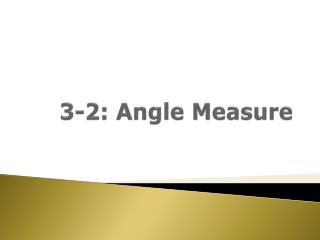DownloadDownload Presentation3-2: Angle Measure

# 3-2: Angle Measure

Download Presentation## 3-2: Angle Measure

- - - - - - - - - - - - - - - - - - - - - - - - - - - E N D - - - - - - - - - - - - - - - - - - - - - - - - - - -
##### Presentation Transcript

1. 3-2: Angle Measure

2. 3-2: Angle Measure • DEGREE: The unit of angle measure Uses the symbol ˚ • PROTRACTOR: A tool for measuring and sketching angles of a given measure.

3. 3-2: Angle Measure • Use a protractor to measure DEF • Place the center middle of the protractor on the angle • Note where the 0 (not the 180) is: top or bottom • Measure the other angle using numbers on the same top/bottom DEF = 125˚

4. 3-2: Angle Measure • Find BXE, CXE, and AXB • BXE = • CXE = • AXB = 115˚ 85˚ 65˚

5. 3-2: Angle Measure • RIGHT ANGLE: An angle with measure of 90˚ • ACUTE ANGLE: An angle with measure between 0˚ & 90˚ • OBTUSE ANGLE: An angle with measure between 90˚ & 180˚

6. 3-2: Angle Measure • Classify each as acute, right, or obtuse angles obtuse right acute

7. 3-2: Angle Measure • The measure for B is 138˚. Solve for x. • 5x – 7 = 138 +7 +7 • 5x = 1455 5 • x = 29

8. 3-2: Angle Measure • Assignment • Worksheet #3-2